# Various approaches used for quantitative study of kinetic processes

| Home | | Biopharmaceutics and Pharmacokinetics |

## Chapter: Biopharmaceutics and Pharmacokinetics : Pharmacokinetics Basic Considerations

The two major approaches in the quantitative study of various kinetic processes of drug disposition in the body.## PHARMACOKINETIC ANALYSIS OF MATHEMATICAL DATA : PHARMACOKINETIC MODELS

Drug movement within the body is a complex process. The major objective is therefore to develop a generalized and simple approach to describe, analyse and interpret the data obtained during in vivo drug disposition studies. The two major approaches in the quantitative study of various kinetic processes of drug disposition in the body are (see Fig. 8.5) –

·            Model approach, and

·            Model-independent approach (also called as non-compartmental analysis).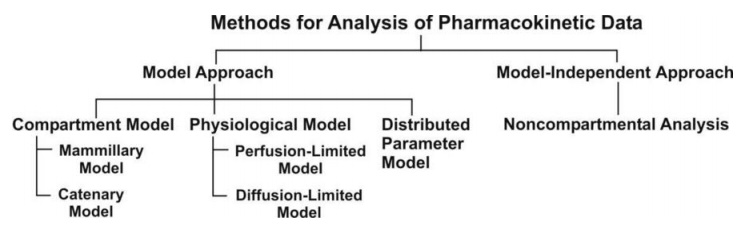Fig. 8.5. Various approaches used for quantitative study of kinetic processes

### A. Pharmacokinetic Model Approach

In this approach, models are used to describe changes in drug concentration in the body with time. A model is a hypothesis that employs mathematical terms to concisely describe quantitative relationships. Pharmacokinetic models provide concise means of expressing mathematically or quantitatively, the time course of drug(s) throughout the body and compute meaningful pharmacokinetic parameters.

Applications of Pharmacokinetic Models

Pharmacokinetic models are useful in —

1.        Characterizing the behaviour of drugs in patients.

2.        Predicting the concentration of drug in various body fluids with any dosage regimen.

3.        Predicting the multiple-dose concentration curves from single dose experiments.

4.        Calculating the optimum dosage regimen for individual patients.

5.        Evaluating the risk of toxicity with certain dosage regimens.

6.        Correlating plasma drug concentration with pharmacological response.

7.        Evaluating the bioequivalence/bioinequivalence between different formulations of the same drug.

8.        Estimating the possibility of drug and/or metabolite(s) accumulation in the body.

9.        Determining the influence of altered physiology/disease state on drug ADME.

10.   Explaining drug interactions.

Caution must however be exercised in ensuring that the model fits the experimental data; otherwise, a new, more complex and suitable model may be proposed and tested.

### Types of Pharmacokinetic Models

Pharmacokinetic models are of three different types –

1. Compartment models are also called as empirical models, and

2. Physiological models are realistic models.

3. Distributed parameter models are also realistic models.

### 1. Compartment Models

Compartmental analysis is the traditional and most commonly used approach to pharmacokinetic characterization of a drug. These models simply interpolate the experimental data and allow an empirical formula to estimate the drug concentration with time.

Since compartments are hypothetical in nature, compartment models are based on certain assumptions

1. The body is represented as a series of compartments arranged either in series or parallel to each other, that communicate reversibly with each other.

2. Each compartment is not a real physiologic or anatomic region but a fictitious or virtual one and considered as a tissue or group of tissues that have similar drug distribution characteristics (similar blood flow and affinity). This assumption is necessary because if every organ, tissue or body fluid that can get equilibrated with the drug is considered as a separate compartment, the body will comprise of infinite number of compartments and mathematical description of such a model will be too complex.

3. Within each compartment, the drug is considered to be rapidly and uniformly distributed i.e. the compartment is well-stirred.

4. The rate of drug movement between compartments (i.e. entry and exit) is described by first-order kinetics.

5. Rate constants are used to represent rate of entry into and exit from the compartment.

Depending upon whether the compartments are arranged parallel or in a series, compartment models are divided into two categories —

·            Mammillary model

·            Catenary model.

### Mammillary Model

This model is the most common compartment model used in pharmacokinetics. It consists of one or more peripheral compartments connected to the central compartment in a manner similar to connection of satellites to a planet (i.e. they are joined parallel to the central compartment). The central compartment (or compartment 1) comprises of plasma and highly perfused tissues such as lungs, liver, kidneys, etc. which rapidly equilibrate with the drug. The drug is directly absorbed into this compartment (i.e. blood). Elimination too occurs from this compartment since the chief organs involved in drug elimination are liver and kidneys, the highly perfused tissues and therefore presumed to be rapidly accessible to drug in the systemic circulation. The peripheral compartments or tissue compartments (denoted by numbers 2, 3, etc.) are those with low vascularity and poor perfusion. Distribution of drugs to these compartments is through blood. Movement of drug between compartments is defined by characteristic first-order rate constants denoted by letter K (see Fig. 8.6). The subscript indicates the direction of drug movement; thus, K12 (K-one-two) refers to drug movement from compartment 1 to compartment 2 and reverse for K21.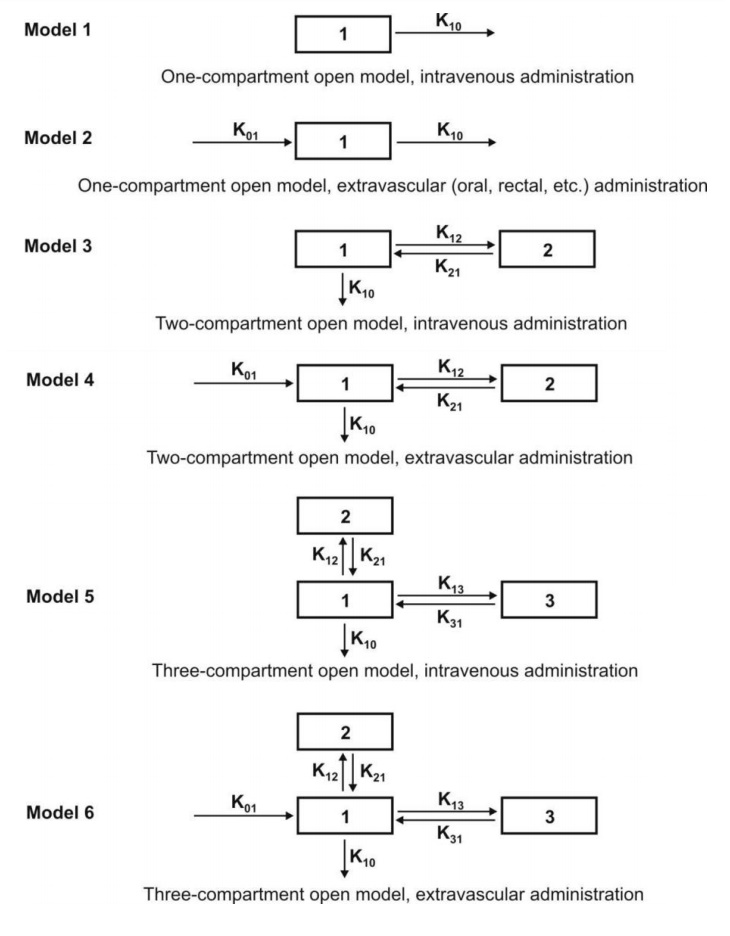Fig. 8.6. Various mammillary compartment models. The rate constant K01 is basically Ka, the first-order absorption rate constant and K10 is KE, the first-order elimination rate constant.

The number of rate constants which will appear in a particular compartment model is given by R.

For intravenous administration, R = 2n – 1 (8.17)

For extravascular administration, R = 2n (8.18)

where n = number of compartments.

### Catenary Model

In this model, the compartments are joined to one another in a series like compartments of a train (Fig. 8.7). This is however not observable physiologically/anatomically as the various organs are directly linked to the blood compartment. Hence this model is rarely used.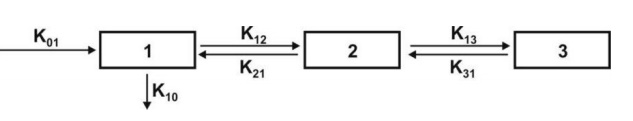Fig. 8.7. A catenary model

The compartment modelling approach has several advantages and applications

1. It is a simple and flexible approach and thus widely used. Fundamentally, the principal use of this approach is to account for the mass balance of drug in plasma, drug in extravascular tissues and the amount of drug eliminated after its administration. It often serves as a ―first-model‖.

2. It gives a visual representation of various rate processes involved in drug disposition.

3. It shows how many rate constants are necessary to describe these processes.

4. It enables the pharmacokineticist to write differential equations for each of the rate processes in order to describe drug-concentration changes in each compartment.

5. It enables monitoring of drug concentration change with time with a limited amount of data. Only plasma concentration data or urinary excretion data is sufficient.

6. It is useful in predicting drug concentration-time profile in both normal physiological and in pathological conditions.

7. It is important in the development of dosage regimens.

8. It is useful in relating plasma drug levels to therapeutic and toxic effects in the body.

9. It is particularly useful when several therapeutic agents are compared. Clinically, drug data comparisons are based on compartment models.

10. Its simplicity allows for easy tabulation of parameters such as Vd, t½, etc.

1. The compartments and parameters bear no relationship with the physiological functions or the anatomic structure of the species; several assumptions have to be made to facilitate data interpretation.

2. Extensive efforts are required in the development of an exact model that predicts and describes correctly the ADME of a certain drug.

3. The model is based on curve fitting of plasma concentration with complex multiexponential mathematical equations.

4. The model may vary within a study population.

5. The approach can be applied only to a specific drug under study.

6. The drug behaviour within the body may fit different compartmental models depending upon the route of administration.

7. Difficulties generally arise when using models to interpret the differences between results from human and animal experiments.

8. Owing to their simplicity, compartmental models are often misunderstood, overstretched or even abused.

Because of the several drawbacks of and difficulties with the classical compartment modelling, newer approaches have been devised to study the time course of drugs in the body. They are — physiological models and noncompartmental methods.

### 2. Physiological Models

These models are also known as physiologically-based pharmacokinetic models (PB-PK models). They are drawn on the basis of known anatomic and physiological data and thus present a more realistic picture of drug disposition in various organs and tissues. The number of compartments to be included in the model depends upon the disposition characteristics of the drug. Organs or tissues such as bones that have no drug penetration are excluded. Since describing each organ/tissue with mathematic equations makes the model complex, tissues with similar perfusion properties are grouped into a single compartment. For example, lungs, liver, brain and kidney are grouped as rapidly equilibrating tissues (RET) while muscles and adipose as slowly equilibrating tissues (SET). Fig. 8.8 shows such a physiological model where the compartments are arranged in a series in a flow diagram.Fig. 8.8. Schematic representation of a physiological pharmacokinetic model. The term Q indicates blood flow rate to a body region. HPT stands for other highly perfused tissues and PPT for poorly perfused tissues. Km is rate constant for hepatic elimination and Ke is first-order rate constant for urinary excretion.

Since the rate of drug carried to a tissue organ and tissue drug uptake are dependent upon two major factors –

·            Rate of blood flow to the organ, and

·            Tissue/blood partition coefficient or diffusion coefficient of drug that governs its tissue permeability,

The physiological models are further categorized into two types –

1. Blood flow rate-limited models These models are more popular and commonly used than the second type, and are based on the assumption that the drug movement within a body region is much more rapid than its rate of delivery to that region by the perfusing blood. These models are therefore also called as perfusion rate-limited models. This assumption is however applicable only to the highly membrane permeable drugs i.e. low molecular weight, poorly ionised and highly lipophilic drugs, for example, thiopental, lidocaine, etc.

2. Membrane permeation rate-limited models These models are more complex and applicable to highly polar, ionised and charged drugs, in which case the cell membrane acts as a barrier for the drug that gradually permeates by diffusion. These models are therefore also called as diffusion-limited models. Owing to the time lag in equilibration between the blood and the tissue, equations for these models are very complicated.

Physiological modelling has several advantages over the conventional compartment modelling –

1.        Mathematical treatment is straightforward.

2.        Since it is a realistic approach, the model is suitable where tissue drug concentration and binding are known.

3.        Data fitting is not required since drug concentration in various body regions can be predicted on the basis of organ or tissue size, perfusion rate and experimentally determined tissue-to-plasma partition coefficient.

4.        The model gives exact description of drug concentration-time profile in any organ or tissue and thus better picture of drug distribution characteristics in the body.

5.        The influence of altered physiology or pathology on drug disposition can be easily predicted from changes in the various pharmacokinetic parameters since the parameters correspond to actual physiological and anatomic measures.

6.        The method is frequently used in animals because invasive methods can be used to collect tissue samples.

7.        Correlation of data in several animal species is possible and with some drugs, can be extrapolated to humans since tissue concentration of drugs is known.

8.        Mechanism of ADME of drug can be easily explained by this model.

1.        Obtaining the experimental data is a very exhaustive process.

2.        Most physiological models assume an average blood flow for individual subjects and hence prediction of individualized dosing is difficult.

3.        The number of data points is less than the pharmacokinetic parameters to be assessed.

4.        Monitoring of drug concentration in body is difficult since exhaustive data is required

Table 8.1 briefly compares features of compartment and physiological models.

TABLE 8.1. Comparison of features of compartment and physiological models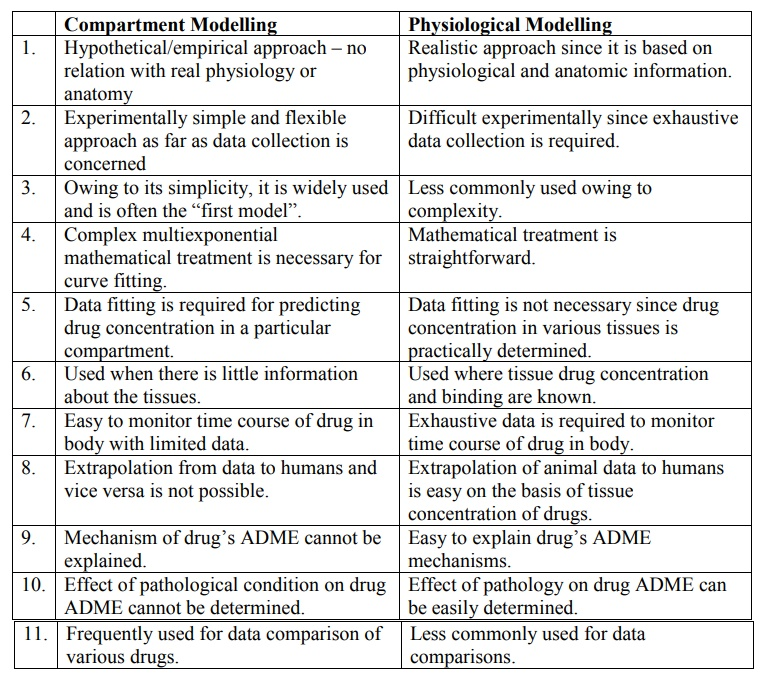Compartment Modelling

1.        Hypothetical/empirical approach – no relation with real physiology or anatomy

2.        Experimentally simple and flexible approach as far as data collection is concerned

3.        Owing to its simplicity, it is widely used and is often the ―first model‖.

4.        Complex multiexponential mathematical treatment is necessary for curve fitting.

5.        Data fitting is required for predicting drug concentration in a particular compartment.

6.        Used when there is little information about the tissues.

7.        Easy to monitor time course of drug in body with limited data.

8.        Extrapolation from data to humans and vice versa is not possible.

9.        Mechanism of drug’s ADME cannot be explained.

10.   Effect of pathological condition on drug ADME cannot be determined.

11.   Frequently used for data comparison of various drugs.

Physiological Modelling

1.        Realistic approach since it is based on physiological and anatomic information.

2.        Difficult experimentally since exhaustive data collection is required.

3.        Less commonly used owing to complexity.

4.        Mathematical treatment is straightforward.

5.        Data fitting is not necessary since drug concentration in various tissues is practically determined.

6.        Used where tissue drug concentration and binding are known.

7.        Exhaustive data is required to monitor time course of drug in body.

8.        Extrapolation of animal data to humans is easy on the basis of tissue concentration of drugs.

9.        Easy to explain drug’s ADME mechanisms.

10.   Effect of pathology on drug ADME can be easily determined.

11.   Less commonly used for data comparisons.

### 3. Distributed Parameter Model

This model is analogous to physiological model but has been designed to take into account –

·            Variations in blood flow to an organ, and

·            Variations in drug diffusion in an organ.

Such a model is thus specifically useful for assessing regional differences in drug concentrations in tumours or necrotic tissues.

The distributed parameter model differs from physiological models in that the mathematical equations are more complex and collection of drug concentration data is more difficult.

### B. Noncompartmental Analysis

The noncompartmental analysis, also called as the model-independent method, does not require the assumption of specific compartment model. This method is, however, based on the assumption that the drugs or metabolites follow linear kinetics, and on this basis, this technique can be applied to any compartment model.

The noncompartmental approach, based on the statistical moments theory, involves collection of experimental data following a single dose of drug. If one considers the time course of drug concentration in plasma as a statistical distribution curve, then:

MRT = AUMC/AUC                  (9.19)

where

MRT = mean residence time

AUMC = area under the first-moment curve

AUC = area under the zero-moment curve

AUMC is obtained from a plot of product of plasma drug concentration and time (i.e. C.t) versus time t from zero to infinity (Fig. 8.9). Mathematically, it is expressed by equation: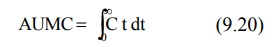AUC is obtained from a plot of plasma drug concentration versus time from zero to infinity. Mathematically, it is expressed by equation: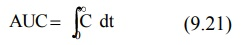Practically, the AUMC and AUC can be calculated from the respective graphs by the trapezoidal rule (the method involves dividing the curve by a series of vertical lines into a number of trapezoids, calculating separately the area of each trapezoid and adding them together).Fig. 8.9. AUC and AUMC plots

MRT is defined as the average amount of time spent by the drug in the body before being eliminated. In this sense, it is the statistical moment analogy of half-life, t½. In effect, MRT represents the time for 63.2% of the intravenous bolus dose to be eliminated. The values will always be greater when the drug is administered in a fashion other than i.v. bolus.

Applications of noncompartmental technique includes

1. It is widely used to estimate the important pharmacokinetic parameters like bioavailability, clearance and apparent volume of distribution.

2. The method is also useful in determining half-life, rate of absorption and first-order absorption rate constant of the drug.

1. Ease of derivation of pharmacokinetic parameters by simple algebraic equations.

2. The same mathematical treatment can be applied to almost any drug or metabolite provided they follow first-order kinetics.

3. A detailed description of drug disposition characteristics is not required.